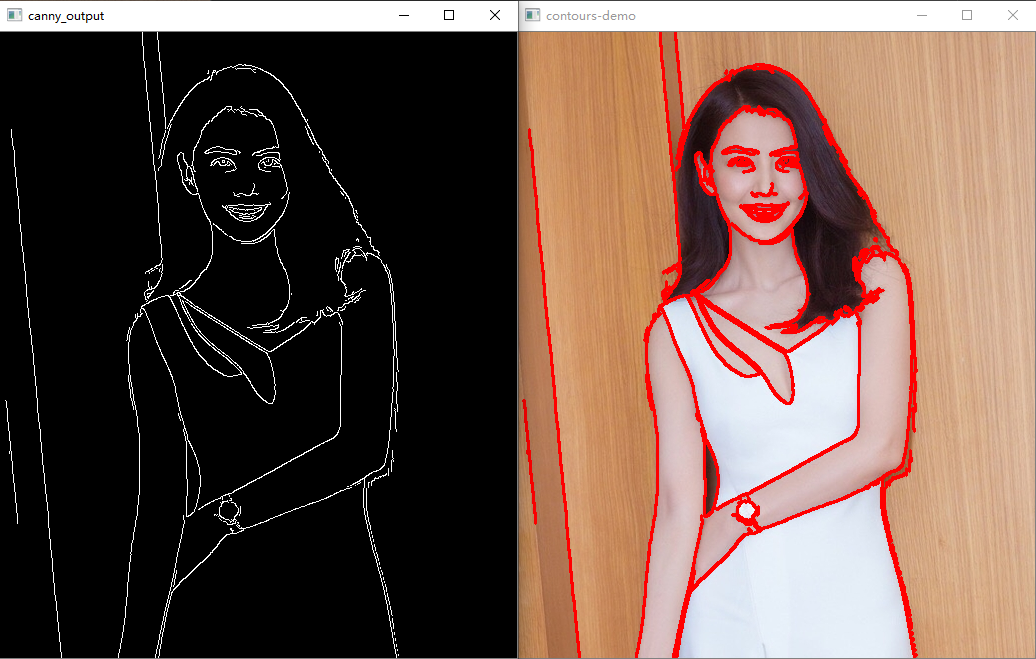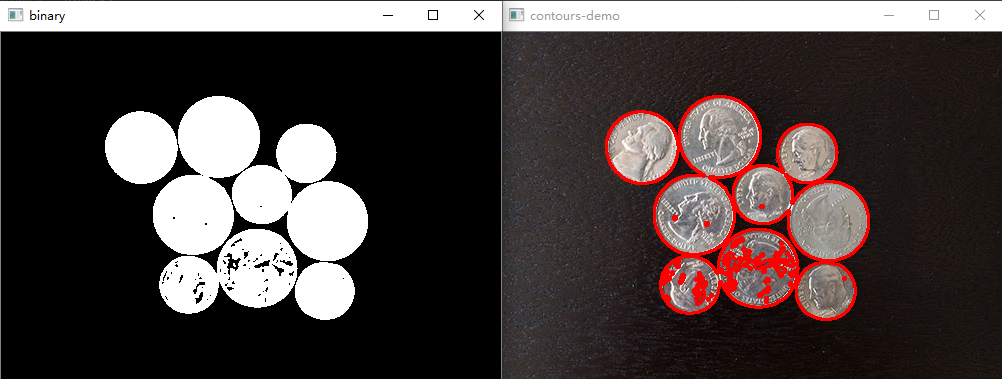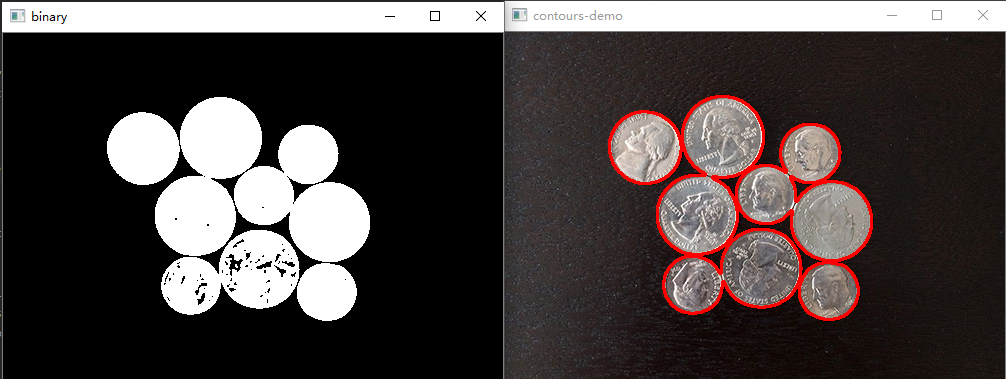## 程序员最近都爱上了这个网站  程序员们快来瞅瞅吧！  it98k网:it98k.com

出租广告位,需要合作请联系站长

+关注

# 48 二值图像分析—轮廓发现

## 代码

``````import cv2 as cv
import numpy as np

def threshold_demo(image):
# 去噪声+二值化
dst = cv.GaussianBlur(image,(3, 3), 0)
gray = cv.cvtColor(dst, cv.COLOR_BGR2GRAY)
ret, binary = cv.threshold(gray, 0, 255, cv.THRESH_OTSU | cv.THRESH_BINARY)
cv.imshow("binary", binary)
return binary

def canny_demo(image):
t = 100
canny_output = cv.Canny(image, t, t * 2)
cv.imshow("canny_output", canny_output)
return canny_output

cv.namedWindow("input", cv.WINDOW_AUTOSIZE)
cv.imshow("input", src)
binary = threshold_demo(src)

# 轮廓发现
contours, hierarchy = cv.findContours(binary, cv.RETR_TREE, cv.CHAIN_APPROX_SIMPLE)
for c in range(len(contours)):
cv.drawContours(src, contours, c, (0, 0, 255), 2, 8)

# 显示
cv.imshow("contours-demo", src)

cv.waitKey(0)
cv.destroyAllWindows()
``````

## 实验结果## 解释

``````contours, hierarchy	=	cv.findContours(image, mode, method[, contours[, hierarchy[, offset]]])
``````

• `Image`表示输入图像，必须是二值图像，二值图像可以threshold输出、Canny输出、inRange输出、自适应阈值输出等。

• `Contours`获取的轮廓，每个轮廓是一系列的点集合

• `Hierarchy`轮廓的层次信息，每个轮廓有四个相关信息，分别是同层下一个、前一个、第一个子节点、父节点

• `mode` 表示轮廓寻找时候的拓扑结构返回

• `RETR_EXTERNAL`表示只返回最外层轮廓
• `RETR_TREE`表示返回轮廓树结构
• `Method`表示轮廓点集合取得是基于什么算法，常见的是基于CHAIN_APPROX_SIMPLE链式编码方法

``````image	=	cv.drawContours(image, contours, contourIdx, color[, thickness[, lineType[, hierarchy[, maxLevel[, offset]]]]])
``````7 0

pdf(new) 更多>